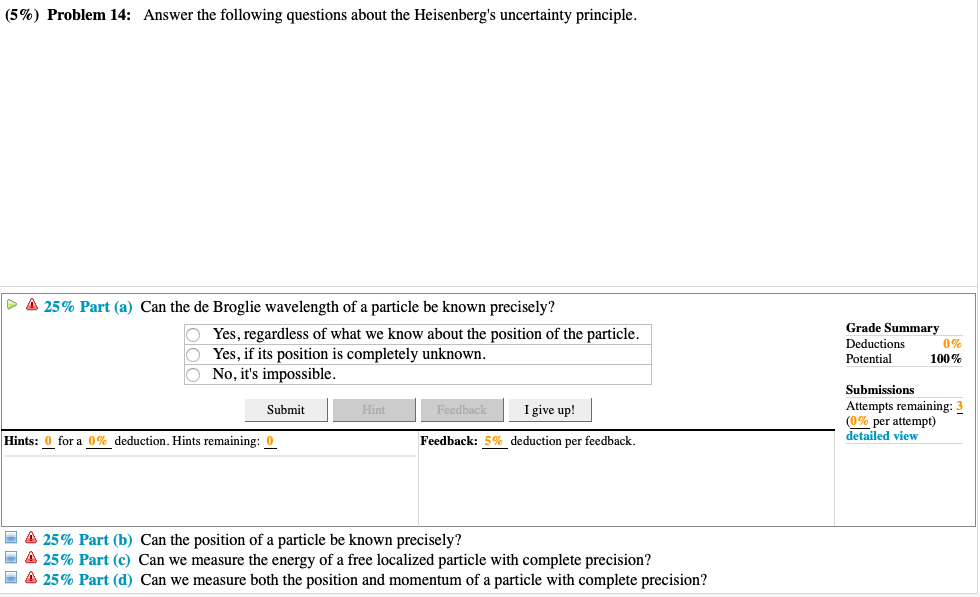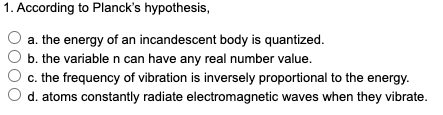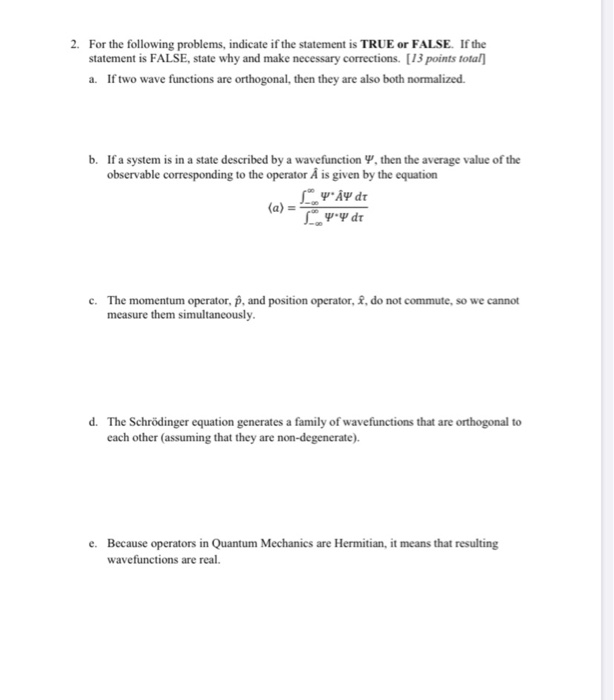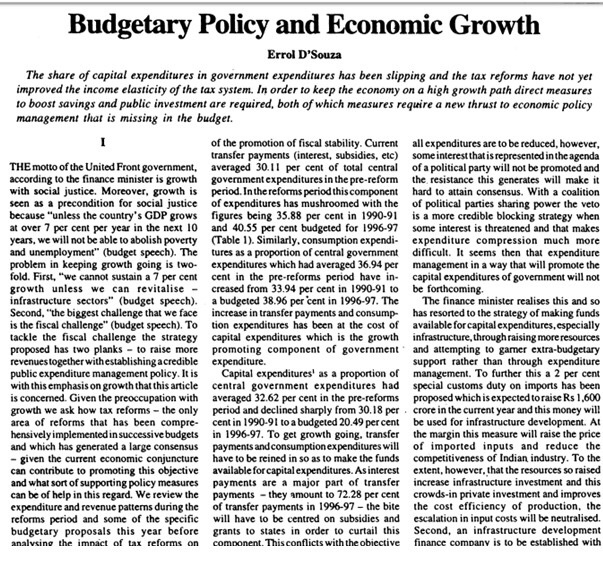Question

# Which of the following are valid regarding the Uncertainty Principle? If a statement is invalid, write...

Which of the following are valid regarding the Uncertainty Principle? If a statement is invalid, write a brief statement indicating why.

(a) It states that you cannot simultaneously measure both the position and momentum of a particle to infinite precision.

(b) It arises from the wave nature of particles.

(c) If the uncertainty in a particle’s momentum is determined to be ∆p, then the maximum uncertainty in its position must be ∆x = ~ 2∆p .

(d) It implies that if a particle decays in a certain time ∆t, then there is a minimum uncertainty in its energy.

(a) Valid

(b) Valid

(c) Invalid; because if uncertainty in momentum is dP ie., it has been determined precisely. Then uncertainty in position of particle must be infinite but here given that it is equal to 2dP, which is wrong.

(d) Invalid; Here nothing can be said about uncertainty in energy of particle if it decays in time dt. We can only have heisenberg's mathematical relationship between these two.

#### Earn Coins

Coins can be redeemed for fabulous gifts.

Similar Homework Help Questions
• ### Select True or False for the following statements about Heisenberg's Uncertainty Principle. It is possible to...

Select True or False for the following statements about Heisenberg's Uncertainty Principle. It is possible to measure simultaneously the y and z positions of a particle exactly. It is not possible to measure simultaneously the x and y momentum components of a particle exactly. It is possible to measure simultaneously the z position and the z momentum component of a particle exactly.

• ### Select True or False for the following statements about Heisenberg's Uncertainty Principle. It is not possible...

Select True or False for the following statements about Heisenberg's Uncertainty Principle. It is not possible to measure simultaneously the x and y positions of a particle exactly. It is not possible to measure simultaneously the x and y momentum components of a particle exactly. It is possible to measure simultaneously the z position and the z momentum component of a particle exactly.

• ### According to the uncertainty principle, it's impossible to exactly measure which two properties of a particle...

According to the uncertainty principle, it's impossible to exactly measure which two properties of a particle simultaneously? Mass and kinetic energy Position and momentum Kinetic energy and momentum Acceleration and position

• ### (5%) Problem 14: Answer the following questions about the Heisenberg's uncertainty principle. Δ 2...(5%) Problem 14: Answer the following questions about the Heisenberg's uncertainty principle. Δ 25% Part (a) Can the de Broglie wavelength of a particle be known precisely? Grade Summary Deductions 0% Potential 100% OYes, regardless of what we know about the position of the particle. O Yes, if its position is completely unknown No, it's impossible. Submissions Attempts remaining: 3 (0% per attempt) detailed view Igive up! Submit Hints: 0 for a deduction. Hints remaining: Feedback: 5% deduction per feedback...

• ### Only asking for accuracy. I'll make sure to leave a like :) 1. According to Planck's...Only asking for accuracy. I'll make sure to leave a like :) 1. According to Planck's hypothesis, a. the energy of an incandescent body is quantized. b. the variable n can have any real number value. c. the frequency of vibration is inversely proportional to the energy. d. atoms constantly radiate electromagnetic waves when they vibrate. 6. Which of the following best describes the nature of light? a. Light has wave properties only. b. Light has particle properties only. c....

• ### 2. For the following problems, indicate if the statement is TRUE or FALSE. If the statement...2. For the following problems, indicate if the statement is TRUE or FALSE. If the statement is FALSE, state why and make necessary corrections. [13 points total] 1. If two wave functions are orthogonal, then they are also both normalized. b. If a system is in a state described by a wavefunction Y, then the average value of the observable corresponding to the operator A is given by the equation YAY dt (a) [ 'ዋ dr c. The momentum operator,...

• ### 12. Which of the following sets of quantum numbers ( n, l, m l,ms) is not...

12. Which of the following sets of quantum numbers ( n, l, m l,ms) is not allowed? a. 2  1  0  + b. 2  2  1 + c. 3  1  0  – d. 5 0  0 + e. 4 2  –1 – 14.  Which of the following atoms is the most electronegative? a. Al b. B c. Cs d. N e. Na 15. The Pauli exclusion principle states that a. an electron can have either particle character or wave character. b. no two electrons in the same atom can have the...

• ### Question 1 Which of the following is an incorrect statement regarding the tax consequences of a...

Question 1 Which of the following is an incorrect statement regarding the tax consequences of a § 306 stock disposition? In a sale of § 306 stock, the shareholder generally recognizes ordinary income equal to the fair market value of the preferred stock on the date it was acquired in the stock dividend. No loss is recognized on a sale of § 306 stock. The issuing corporation’s E & P is not reduced by a sale of § 306 stock....

• ### I need Summary of this Paper i dont need long summary i need What methodology they used , what is the purpose of this p...I need Summary of this Paper i dont need long summary i need What methodology they used , what is the purpose of this paper and some conclusions and contributes of this paper. I need this for my Finishing Project so i need this ASAP please ( IN 1-2-3 HOURS PLEASE !!!) Budgetary Policy and Economic Growth Errol D'Souza The share of capital expenditures in government expenditures has been slipping and the tax reforms have not yet improved the income...